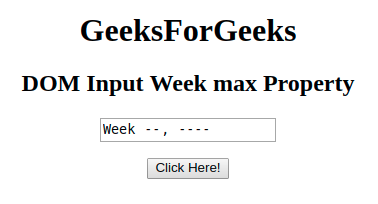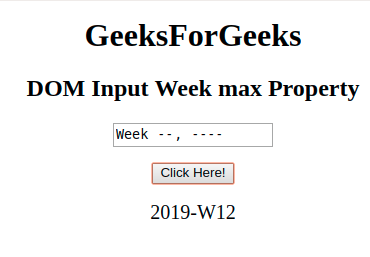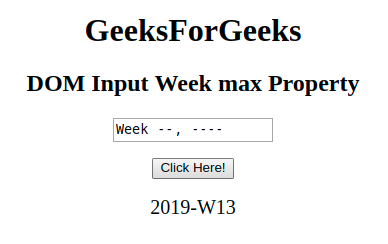# HTML | DOM Input Week max Property

The DOM Input Week max property in HTML DOM is used to set or return the value of the max attribute of a week field. The max attribute specifies the maximum value (week and year) for a week field.

Syntax:

• It returns the max property.
`weekObject.max`
• It is used to set the max property.
`weekObject.max = YYYY-WWW`

Property Values:

• YYYY-WWW: This values states the maximum year and week. Here YYYY is the year i.e. 2019 and WWW is the week with starting letter W i.e. W32 .

Return Value: It returns a string which represents the maximum value allowed for a week field.

Example-1: This example returns the Input Week max property.

## HTML

 ` ` `<``html``> ` `<``head``> ` `    ``<``title``> ` `        ``HTML DOM Input Week max Property` `    `` ` ` ` `<``body` `style="text-align:center;"> ` `    ``<``h1``>GeeksForGeeks ` `    ``<``h2``>DOM Input Week max Property ` `    ``<``form` `id="myGeeks">` `        ``<``input` `type="week" id="week_id" name="geeks" max="2019-W12"> ` `    ``` `    ``<``br``>` `    ``<``button` `onclick="myGeeks()">Click Here! ` `    ``<``p` `id="GFG" style="font-size:20px;"> ` `    `  `    ``` `    ``<``script``> ` `        ``function myGeeks() { ` `            ``var gfg = document.getElementById("week_id").max;` `            ``document.getElementById("GFG").innerHTML = gfg;` `        ``} ` `    `` ` ` ` ``

Output:

Before clicking on the button:After clicking on the button:Example-2: This Example illustrates how to set the property.

## HTML

 ` ` `<``html``> ` `<``head``> ` `    ``<``title``> ` `        ``HTML DOM Input Week max Property` `    `` ` ` ` `<``body` `style="text-align:center;"> ` `    ``<``h1``>GeeksForGeeks ` `    ``<``h2``>DOM Input Week max Property ` `    ``<``form` `id="myGeeks">` `        ``<``input` `type="week" id="week_id" name="geeks"> ` `    ``` `    ``<``br``>` `    ``<``button` `onclick="myGeeks()">Click Here! ` `    ``<``p` `id="GFG" style="font-size:20px;"> ` `    `  `    ``` `    ``<``script``> ` `        ``function myGeeks() { ` `            ``var gfg = document.getElementById("week_id");` `            ``gfg.max = "2019-W13";` `            ``var g =    gfg.max;        ` `            ``document.getElementById("GFG").innerHTML = g;` `        ``} ` `    `` ` ` ` ``

Output:

Before clicking on the button:After clicking on the button:Supported Browsers: The browser supported by DOM input Week max Property are listed below: